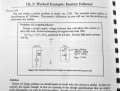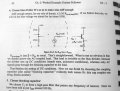# The Art of Electronics Student Manual Emitter Follower question

#### elpaso

Joined Feb 9, 2013
1
Hi,

I'm a true beginner and I'm trying to learn electronics from the book(s) cited in the topic.

I have a question about the worked example emitter-follower at page 90-92 of the student manual.

I'm attaching the relevant pages.My question is about the divider R1 and R2. Following the text, the desired Rth of the divider should be 10 times less than Rin (R looking into the base), which in turn is about Hfe * RE, so Rth of the divider should be about 150K.

If R1||R2 must be = Rth = 150K, I would say that R1=R2=300K.

But the value chosen in the text for R1 and R2 is 270K: why?
Is that just an approximation to the lower standard resistor value or is there anything wrong in my calculation?

#### Audioguru

Joined Dec 20, 2007
11,248
If R1||R2 must be = Rth = 150K, I would say that R1=R2=300K.

But the value chosen in the text for R1 and R2 is 270K: why?
Is that just an approximation to the lower standard resistor value or is there anything wrong in my calculation?